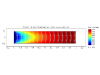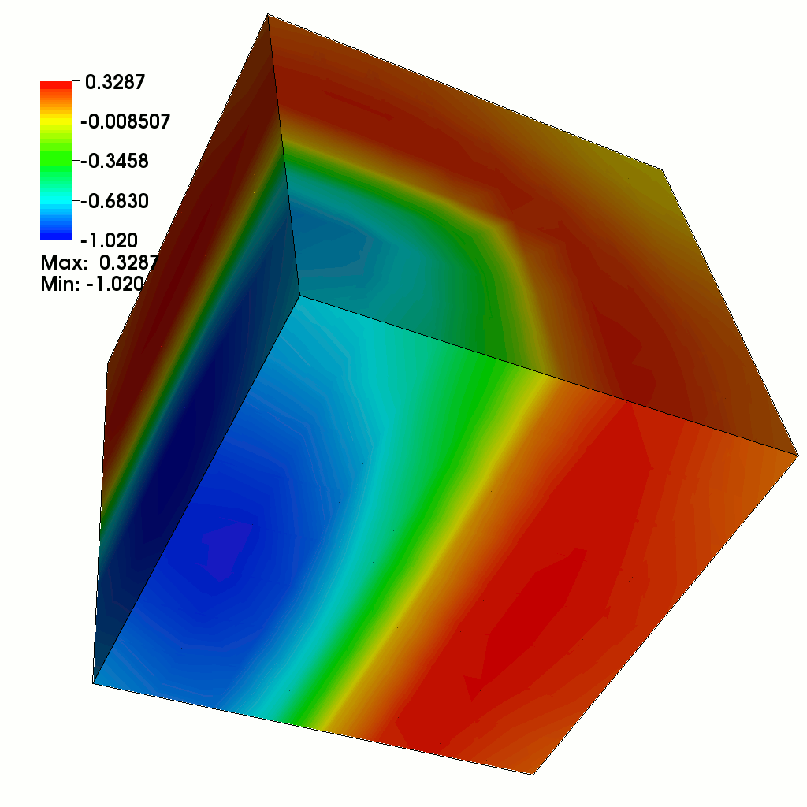Research Group Computational Methods in Systems and Control Theory

Diese Seite wird nicht mehr aktualisiert. Bitte besuchen Sie unsere neue Webpräsenz.

# Theses Topics

## Thesis Topic: Diplom/Master Thesis

October 19, 2013Structure-Preserving Balancing of Matrix Pencils Arising in Linear-Quadratic Optimal Control
Balancing is a popular method to increase the accuracy when numerically computing the eigenvalues of a matrix. The aim of this work is the development of an algorithm that enables the balancing of even or skew-Hamiltonian/Hamiltonian matrix pencils by using structure-preserving transformations. This is necessary to be able to use structure-exploiting algorithms to compute the eigenvalues of the balanced pencils. The algorithm should be implemented in FORTRAN 77 and tested for some benchmark examples.
Contact: Matthias Voigt

## Thesis Topic: Diplom/Master Thesis

October 19, 2013Fast Numerical Computation of Structured Real Stability Radii for Large-Scale Matrices and Pencils
Stability radii are a measure for the robustness of differential or differential-algebraic equations under perturbations. The goal of this project is to adapt existing methods in order to compute the structured real stability radius by using an optimization procedure over structured pseudospectra.
Contact: Matthias Voigt

## Thesis Topic: Diplom/Master Thesis

December 18, 2012A CG-Method with ADI preconditioning for the solution of large sparse Lyapunov equations with symmetric coefficient matrices
Contact: Jens Saak

## Thesis Topic: Diplom/Master Thesis

December 18, 2012Step size control for the numerical solution of large and sparse matrix differential Riccati equations
Contact: Jens Saak

## Thesis Topic: Diplom/Master Thesis

January 24, 2012Kalman-Yakubovich-Popov Lemma for Algebraic Difference Equations
In many fields of systems and control theory, dynamical systems are analyzed via certain rational matrix functions (so-called Popov functions). Properties of Popov functions can often be characterized via solvability of linear matrix inequalities. The aim of this thesis is the adaption of the existing theory to the case that the dynamical system is given as algebraic difference equation. Furthermore, relations to the structure of palindromic matrix pencils should be analyzed. Optionally, applications of the theory can be taken into account, e.g., for the structure analysis of dynamical systems or optimal control.
Contact: Matthias Voigt

## Thesis Topic: Diploma/Master Thesis

January 23, 2012Compute the subgradient of the spectral abscissa numerically.
Contact: Sara Grundel

## Thesis Topic: Diplom/Master Thesis:

January 18, 2012Numerical solution of low-rank representations of band-limited Gramians
In this thesis we investigate the representation of band-limited Gramians by matrix equations and the low-rank approximation of thereof.
Contact: Patrick Kürschner

## Thesis Topic: Diplom/Master Thesis:

July 07, 2011Regulator based tracking control of a parabolic partial differential equation under control constraints
We are searching for a regulator based feedback control u. Since this is not covered by the linear quadratic regulator approach, we first compute a reference pair of control and solution trajectory via open loop optimization. The resulting pair is then tracked by the regulator based feedback control.
Contact: Jens Saak

## Thesis Topic: Diplom/Master ThesisDevelopment and implementation of a Multigrid-ADI method for solving Lyapunov equations

## Thesis Topic: Diplom/Master ThesisComputation of Lyapunov exponents for dynamical systems

## Thesis Topic: Diplom/Master ThesisDevelopment and comparison of model reduction methods for concrete applications (several topics, possibility to work in a group)

May 08, 2013

## Thesis Topic: Bachelor Thesis

December 18, 2012Model order recuction using balanced truncation for differential algebraic second order systems in M.E.S.S.
Contact: Jens Saak

## Thesis Topic: Bachelor Thesis

December 18, 2012Numerical Solution of large and sparse matrix equations on distributed memory parallel machines
Contact: Jens Saak

## Thesis Topic: Bachelor Thesis

December 18, 2012Approximate solution of the Linear systems of equations in the ADI Method for large and sparse matrix equations
Contact: Jens Saak

## Thesis Topic: Bachelor Thesis

December 18, 2012Numerical solution of large sparse Matrix equations on CUDA GPUs
Contact: Jens Saak

## Thesis Topic: Bachelor Thesis

December 18, 2012Numerical solution of large and sparse matrix equations in Python
Contact: Jens Saak

## Thesis Topic: Bachelor/Diploma/Master Thesis

January 23, 2012Model reduction in complex biochemical networks
An important tool in the analysis of complex physical phenomena is the simulation of the underlying mathematical models, which are often given by systems of ordinary and/or partial differential equations. As one is interested in models as accurate as possible, linear models are often insufficient such that one is faced with large-scale nonlinear systems. Frequently, these cannot be handled efficiently, necessitating model order reduction, i.e., the construction of a smaller system approximating the original one. In this thesis, a recently introduced approach for nonlinear model reduction should be implemented and tested by means of a real-life application arising in the context of biochemical reaction networks.
Contact: Tobias Breiten

## Thesis Topic: Bachelor Thesis

January 19, 2012This thesis deals with the study and implementation of shooting methods for PDE-constrained optimization problems. This approach will combine the numerical analysis of ODEs with efficient techniques used in numerical linear algebra.
Contact: Martin Stoll

## Thesis Topic: Bachelor Thesis

January 19, 2012Optimal Control of a second order partial differential equation with acceleration measurements. Optimal control problems for infinite dimensional second order systems with acceleration measurements are in general ill-posed. Jacob and Morris proposed a method exploiting the actual implementation of the measurement that pulls the problem back to a well posed one. Here such a system is to be implemented and solved numerically.
Contact: Jens Saak

## Thesis Topic: Bachelor Thesis

January 18, 2012Simultaneous iterative solution of the adjoint linear systems in the dual low-rank ADI iteration for Lyapunov equations
Solving dual large-scale Lyapunov equations is a main step for carrying out balanced truncation model order reduction for linear, time-invariant control systems. Both Lyapunov equation can be solved simultaneously in one run of the dual low-rank ADI iteration. Here we are going to investigate the simultaneous iterative solution of the occurring adjoint linear systems with BiCG / QMR.
Contact: Patrick Kürschner
Top
Jens Saak, jens.saak@mathematik.tu-chemnitz.de